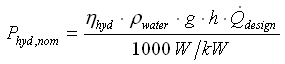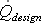# HOMER Pro 3.14Type: Output Variable Units: kW Symbol: Phyd,nom

The nominal hydro power is the nominal power of the hydro system, or the power produced by the hydro turbine given the available head and a stream flow equal to the design flow rate of the hydro turbine. The calculation of the nominal hydro power includes the efficiency of the hydro turbine, but not the pipe head loss.

Note: HOMER uses this value only to identify the size of the hydro system and allow easy comparison to the sizes of other Components of the power system.

HOMER calculates the nominal hydro power using the following equation:where: Phyd,nom = nominal power output of the hydro turbine [kW] ηhyd ρwater = density of water [1000 kg/m3] g = acceleration due to gravity [9.81 m/s2] h = available head [m]= the design flow rate of the hydro turbine [m3/s]